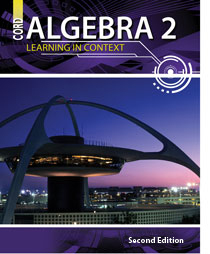# Algebra 2 - 2nd Edition

## Chapter 4: Solving Quadratic Equations That Have Complex Solutions

Some links are repeated for use with more than one lesson.

### These links are for use with the entire chapter.

This page contains an interactive tutorial on quadratic equations. Pass mouse over each colored area to reveal.

### 4.1 Solving Quadratic Equations by Graphing

http://www.teachertube.com/viewVideo.php?video_id=20844

http://www.ronblond.com/M11/QFA.sf.APPLET/index.html
This page contains an applet for graphing quadratic equations and see the solution. This website requires Java to run some of its features.

### 4.2 Solving Quadratic Equations Using Square Roots

This page contains several quadratic equation solvers with step-by-step instructions, including one that uses the square root method.

### 4.3 Solving Quadratic Equations by Completing the Square

http://www.mathsisfun.com/algebra/completing-square.html

This series of pages (Click Next) contains a tutorial on completing the square and shows how the quadratic formula is derived.

### 4.4 Solving Quadratic Equations by Factoring

http://www.regentsprep.org/regents/math/algtrig/ATE3/indexATE3.htm

This series of pages contains a detailed review of solving quadratic equations by factoring.